×

Search anything:

# Polygon Triangulation

#### computational geometry AlgorithmsGet this book -> Problems on Array: For Interviews and Competitive Programming

In this article, we have explained the problem statement of Polygon Triangulation along with algorithmic approaches.

• Introduction
• Mathematical Approach
• Algorithmic Analysis
• Implementation
• Overview

# Introduction

Within computational geometry, we can use polygons as the building blocks to many algorithms. A polygonal curve is a finite chain of line segments, these line segments are called edges and their endpoints called vertices. Using this we can define a simple polygon as a closed polygonal curve with no self-intersection.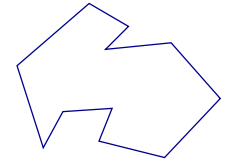There are numerous topologically different polygons, including convex, monotone, star-shaped or polygons with holes, some can be seen below: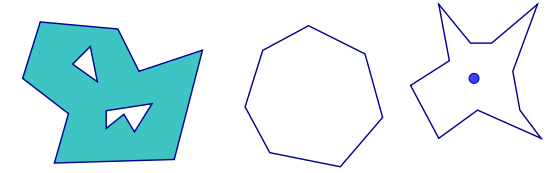Polygons have unique properties that make them suitable for algorithmic use:

• Flexible, able to model arbitrarily complex shapes
• Efficient, produce simple algorithms with an algebraic representation
This leads to polygons being more powerful in algorithm use than other shapes, for example circles or rectangles.

Doing so will reduce a complex shape into a collection of simple ones. This is the first step in many advanced algorithms. Polygonal triangluation finds use in cases such as robotics motion planning, computer vision, mesh generation and more.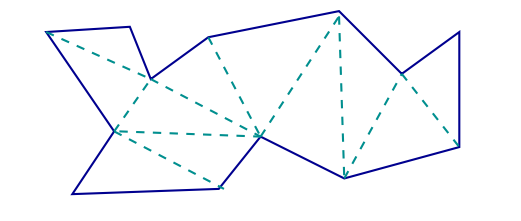# Approach

• Every simple polygon can be triangulated
• Every triangulation of a polygon with n number of sides contains exactly n-2 triangles.

For example:The above polygon has 13 vertices, therefore 11 triangles are produced.

To further demonstrate the use of polygon triangulation, we shall examine a classic computer science problem, the art gallery problem. This problem states that given a gallery in the shape of a polygon with N-vertices, what is the minimum amount of guards that can be placed within the polygon or around its edges so that they can observe the entire gallery. The guards cannot move from the vertices they are placed and have a 360 degree field of view, meaning we can deduct they can see entirely around them as long as an edge does not obstruct their vision.

We can see a visualisation of the guards' field of view:We can find the right number of guards for said polygon using triangulation: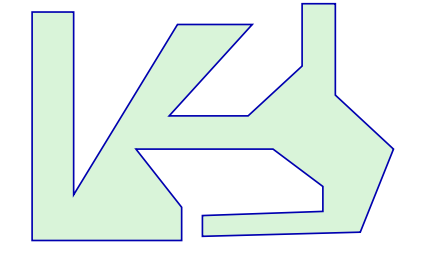Our art gallery has 22 vertices, the art gallery theorem states that every n-gon can be guarded with n/3 guards at vertices. It also mentions that some n-gons require at least n/3 guards (arbitrary).

In our case that means that the number of guards required for our polygon is 7 as 22/3 equals around 7.33To prove this generally, we can say that the triangulation of polygons can be 3-coloured.

• Polygon + triangulation is a planar graph
• 3-coloured: Each vertex can be labeled 1, 2 or 3 with no edge or diagonal having the both its endpoints with the same label.
1. Remove an ear from the polygon
2. 3 colour the rest
3. Return the ear, colouring the new vertex using the label not used by the boundary diagonal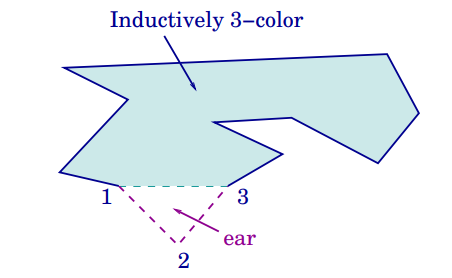To show this works for an example: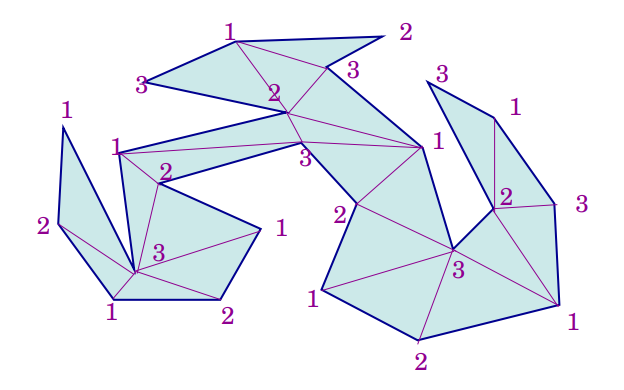• Triangulate the polygon P and 3-colour it
• The least frequent colour appears at most n/3 times
• Place the guards at this colour's positions, each triangle has all three colours so can be seen by a guard

Here we can see that 3 appears the least with 7 times. Therefore we need 7 guards.

# Algorithm Analysis

There are numerous ways that we can approach polygon triangulation in an algorithm, for example, a naive implementation would be to check n^2 choices for a diagonal n-1 times, with each check taking O(n) giving us an O(n^4) complexity. Another naive method would be to find the ears of the polygon recursively, taking O(n) each meaning that the total algorithm is O(n^2). These are still not very efficient, there is a randomized algorithm that would produce this in linear time however it is very complicated and not always reliable therefore we can settle on a solution that turns out to be O(nlogn).

To do this we should:

• Partition the polygon into trapezoids
• Convert trapezoids to monotone subdivisons
• Triangulate each division

We say that a polygon chain C is monotone with respect to line L if any line orthogonal to L intersects C in at most one point. A polygon is monotone with respect to L if it can be decomposed into 2 monotone chains with respect to L. In this case, line L is the x-axis.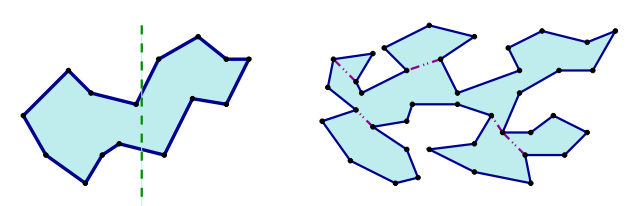To partition the polygon, at each vertex, extend a vertical line until it hits an edge, each face is a trapezoid, which may degenerate into a triangle. This has a complexity of O(nlogn).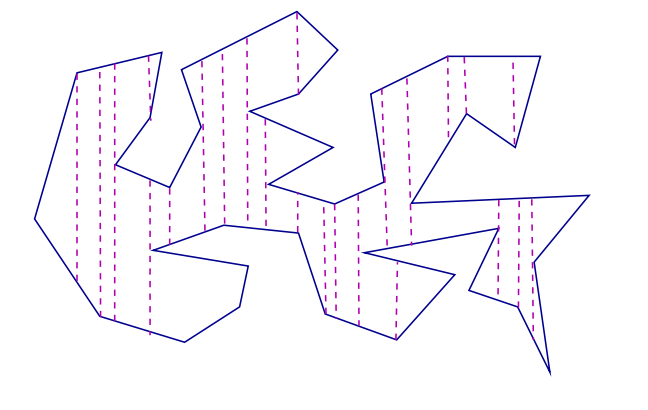To then conduct monotone subdivision:

• Call a reflex vertex with both rightward edges, split, or both leftward edges, merge.
• These vertices are what create non-monotonicity, therefore we should add a diagonal to each to remove it.
• To each split, add a diagonal which joins it to the polygon vertex of its left, for merges, their right.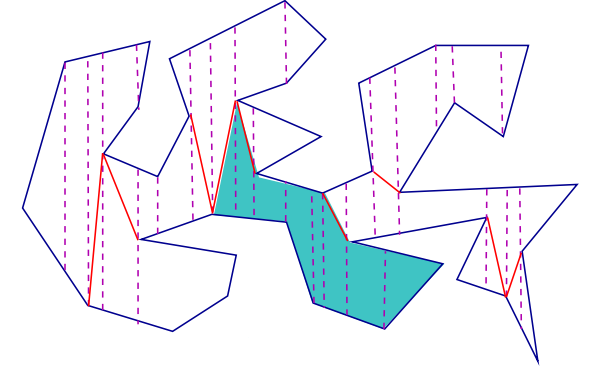• Assume that decomposition to be represented by a doubly-connected edge list.
• Matching split and merge vertices takes O(1) time.
• By removing all trapezoidal edges, the polygon boundary + new split/merge edges form the subdivisions.

The final stage in our algorithm is the actual triangulation, the pseuodcode for this could be written as such:

``````<v1, v2,...,vn> sorted (left to right)
v1, v2 pushed to stack
for i=3 to n do
if vi & top(stack) on same chain then
add diagonal vi,vj,...,vi,vk where vk is last to give legal diagonal
pop vj,...,vk-1 -> push vi
else do
add diagonals from vi to all vertices in stack, then pop them
save vtop, push vtop and vi
``````

To show this in process, see the diagram below: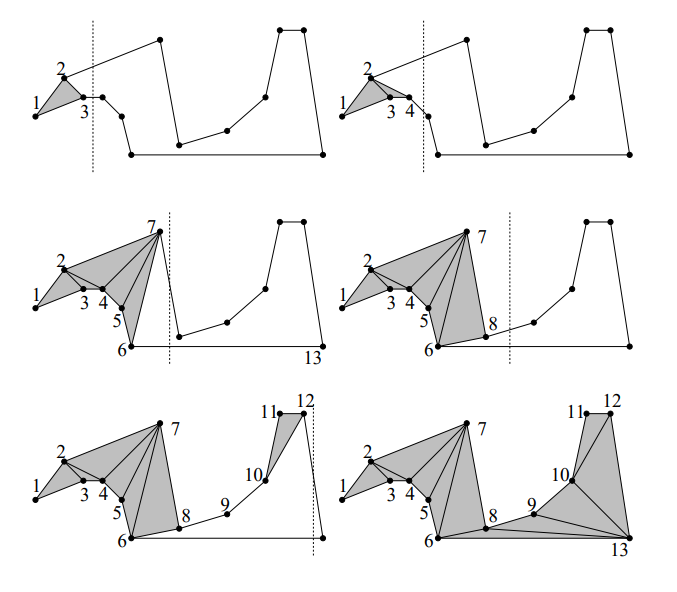# Implementation

Below is an implementation of this in C++:

Showing triangulate.h:

``````#ifndef TRIANGULATE_H
#define TRIANGULATE_H
#include <vector>

class Vector2d
{
public:
Vector2d(float x,float y)
{
Set(x,y);
};

float GetX(void) const { return mX; };

float GetY(void) const { return mY; };

void  Set(float x,float y)
{
mX = x;
mY = y;
};
private:
float mX;
float mY;
};

typedef std::vector< Vector2d > Vector2dVector;

class Triangulate
{
public:

static bool Process(const Vector2dVector &contour,
Vector2dVector &result);

// compute area of a contour/polygon
static float Area(const Vector2dVector &contour);

static bool InsideTriangle(float Ax, float Ay,
float Bx, float By,
float Cx, float Cy,
float Px, float Py);

private:
static bool Snip(const Vector2dVector &contour,int u,int v,int w,int n,int *V);

};

#endif
``````

Then using triangulate.h in a .cpp file:

``````/*After defining triangulate.h, we then can implement it in another file*/
#include <stdio.h>
#include <stdlib.h>
#include <string.h>
#include <assert.h>

#include "triangulate.h"

static const float EPSILON=0.0000000001f;

float Triangulate::Area(const Vector2dVector &contour)
{

int n = contour.size();

float A=0.0f;

for(int p=n-1,q=0; q<n; p=q++)
{
A+= contour[p].GetX()*contour[q].GetY() - contour[q].GetX()*contour[p].GetY();
}
return A*0.5f;
}

bool Triangulate::InsideTriangle(float Ax, float Ay,
float Bx, float By,
float Cx, float Cy,
float Px, float Py)

{
float ax, ay, bx, by, cx, cy, apx, apy, bpx, bpy, cpx, cpy;
float cCROSSap, bCROSScp, aCROSSbp;

ax = Cx - Bx;  ay = Cy - By;
bx = Ax - Cx;  by = Ay - Cy;
cx = Bx - Ax;  cy = By - Ay;
apx= Px - Ax;  apy= Py - Ay;
bpx= Px - Bx;  bpy= Py - By;
cpx= Px - Cx;  cpy= Py - Cy;

aCROSSbp = ax*bpy - ay*bpx;
cCROSSap = cx*apy - cy*apx;
bCROSScp = bx*cpy - by*cpx;

return ((aCROSSbp >= 0.0f) && (bCROSScp >= 0.0f) && (cCROSSap >= 0.0f));
};

bool Triangulate::Snip(const Vector2dVector &contour,int u,int v,int w,int n,int *V)
{
int p;
float Ax, Ay, Bx, By, Cx, Cy, Px, Py;

Ax = contour[V[u]].GetX();
Ay = contour[V[u]].GetY();

Bx = contour[V[v]].GetX();
By = contour[V[v]].GetY();

Cx = contour[V[w]].GetX();
Cy = contour[V[w]].GetY();

if ( EPSILON > (((Bx-Ax)*(Cy-Ay)) - ((By-Ay)*(Cx-Ax))) ) return false;

for (p=0;p<n;p++)
{
if( (p == u) || (p == v) || (p == w) ) continue;
Px = contour[V[p]].GetX();
Py = contour[V[p]].GetY();
if (InsideTriangle(Ax,Ay,Bx,By,Cx,Cy,Px,Py)) return false;
}

return true;
}

bool Triangulate::Process(const Vector2dVector &contour,Vector2dVector &result)
{

int n = contour.size();
if ( n < 3 ) return false;

int *V = new int[n];

if ( 0.0f < Area(contour) )
for (int v=0; v<n; v++) V[v] = v;
else
for(int v=0; v<n; v++) V[v] = (n-1)-v;

int nv = n;

int count = 2*nv;

for(int m=0, v=nv-1; nv>2; )
{
if (0 >= (count--))
{
return false;
}

int u = v  ; if (nv <= u) u = 0;
v = u+1; if (nv <= v) v = 0;
int w = v+1; if (nv <= w) w = 0;

if ( Snip(contour,u,v,w,nv,V) )
{
int a,b,c,s,t;

a = V[u]; b = V[v]; c = V[w];

result.push_back( contour[a] );
result.push_back( contour[b] );
result.push_back( contour[c] );

m++;

for(s=v,t=v+1;t<nv;s++,t++) V[s] = V[t]; nv--;

count = 2*nv;
}
}

delete V;

return true;
}
``````

# Overview

In this article at OpenGenus, we have covered polygon triangulation, its uses, solved a problem using it, proved it through induction and then demonstrated how to apply it in algorithmic form and finally, showing a C++ implementation of the algorithm, first writing a header file and then using it in for implementation, ready to be used within a class or similar.